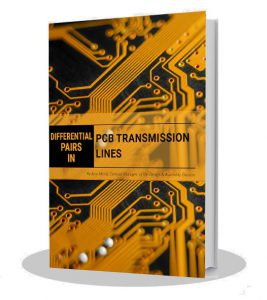# Differential Pairs in PCB Transmission Lines: The Physical Parameters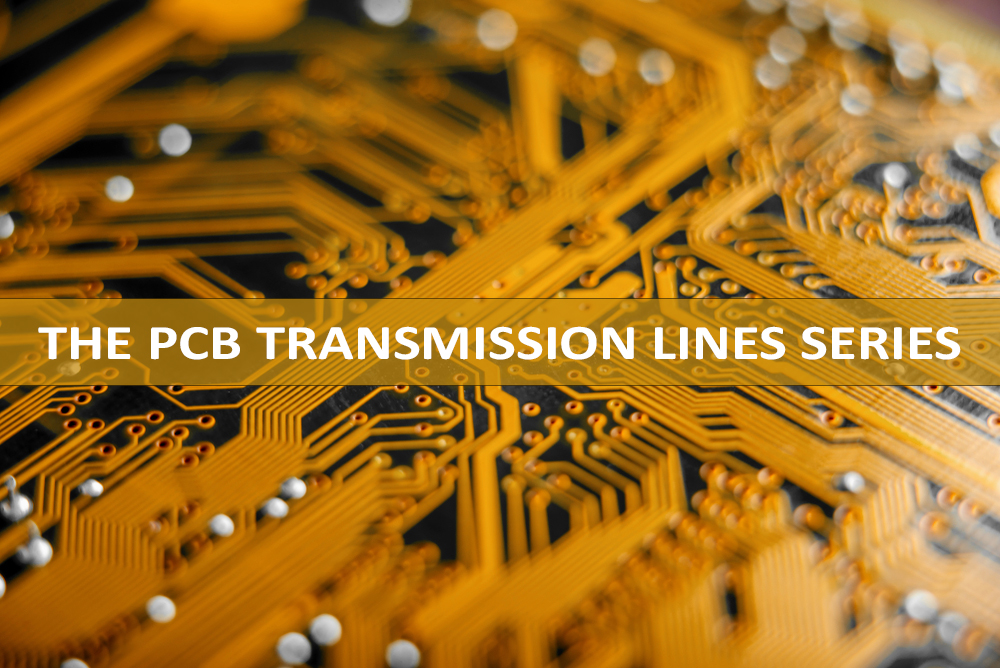After reviewing the differential impedance as well as the even or common mode, let’s now dive into the physical parameters of a differential pair.

• Line inductances and capacitances
• Case 1: Odd mode
• Case 2: Even mode
• Single-ended impedance
• Two crosstalk related parameters

### Detailed analysis of a differential pair in terms of line inductances and capacitances

The analysis of a lossless single-ended transmission line was done using the following circuit model for an infinitesimally small line of length ‘delta x’: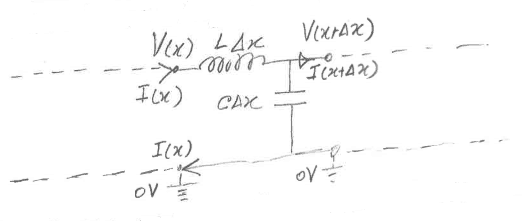Here, ‘L’ and ‘C’ are respectively the inductance and capacitance per unit length of the line. After analysis, we had derived that the characteristic (or instantaneous) impedance of the line at a point was given by: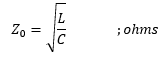And the propagation delay ‘Pd’ was given by: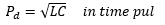We can now apply the above model and results to the analysis of the lines of a differential pair in terms of inductances and capacitances involved. And we are assuming that lines as such that the conductor resistances (‘R’s’) and dielectric conductances (‘G’s’) can be neglected for the impedance and propagation delay purposes. This will be the case at practical frequencies of interest.

The following diagram gives the circuit model of an infinitesimally small length of a differential pair line: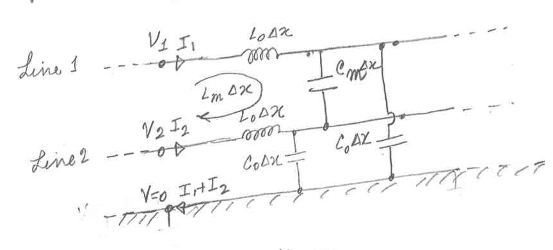Here ‘L0’ and ‘C0’ are respective by the inductance and capacitance of each line per unit length. ‘Lm’ is the mutual inductance per unit length between line 1 and line 2. ‘Cm’ is the capacitance per unit length between line 1 and line 2.

### Case 1: Odd mode (purely differential signal)

Here ‘V2 = -V1’ and ‘I2 = -I1’. Thus, no current flows in the return path. ‘Cm delta x’ can be considered as two capacitors, each of value ‘2Cm delta x’, in series whose center point is at zero potential. (That is because of the potential division between two equal capacitors.) Thus, the equivalent circuit of above in odd mode becomes: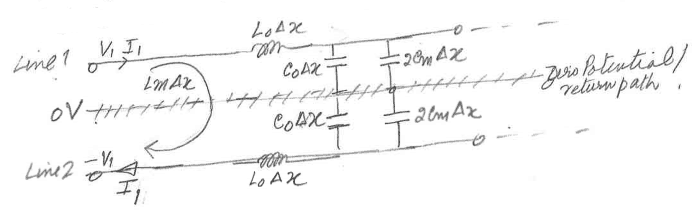Let’s look at line 1 (the line 2 case is exactly similar).

The inductive voltage in line 1 will comprise of two parts. One due to ‘I’, flowing through ‘L0 delta x’. The other one due to ‘I2 = -I1’, flowing through ‘Lm delta x’. These can be equivalently stated as due to ‘I’, flowing through ‘(L0 – Lm) delta x’. Thus, the effective inductance per unit length of line 1 in odd mode, denoted by ‘Lodd’, will be given by: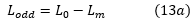And the effective capacitance between line 1 and zero potential line is ‘(C0 + 2Cm) delta x’. Thus, the odd mode line capacitance per unit length is: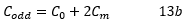By definition and similar to results derived in the case of the single-ended line, the odd mode characteristic impedance is given by: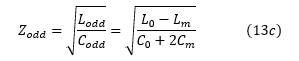And the propagation delay per unit length for odd mode signals is given by: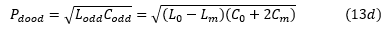This is the propagation delay per unit length that will take place for purely the differential part of the signal. The point to also remember here is that the odd mode or purely differential signal’s electromagnetic waves exist largely in and around the space between the two lines. And they are relatively less affected by the reference or ground planes.

### Case 2: Even mode (purely common mode signal)

In this case, ‘V2 = V1’ and as the two lines of the pair are identical, ‘I1 = I2’. Since ‘V1 = V2’, the capacitance ‘Cm’ between the two lines will have no effect on the currents in the two lines. It can therefore be ignored, leading to the following equivalent circuit: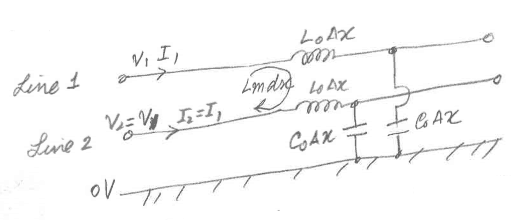Let’s look at line 1 (the line 2 case is identical).

The inductive voltage in line 1 will be contributed by ‘I1’, flowing in ‘L0 delta x’. And by ‘I2 = I1’’, flowing in ‘Lm delta x’. This is equivalent to saying ‘I1’ flowing through ‘(L0 + Lm) delta x’. Therefore, the effective inductance per unit length of either line in even mode will be: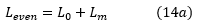And the effective capacitance per unit length of either line in even mode will be: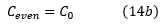Therefore, the even mode impedance of either line will be given by: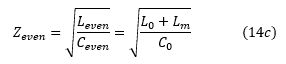And the propagation delay per unit length for even mode signals is given by: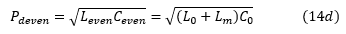Equations (13c) and (14c) clearly indicate that ‘Zeven’ is greater than ‘Zodd’. From theory, it is also known that ‘Lm’ is lesser than ‘L0’ and ‘Cm’ is lesser than ‘C0’.

### ‘Zse’, ‘Zm’ and ‘K’ in terms of ‘L0’, ‘C0’, ‘Lm’, ‘Cm’

Using equations (12), (13) and (14), we have: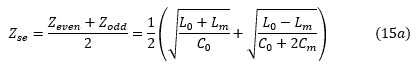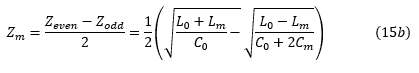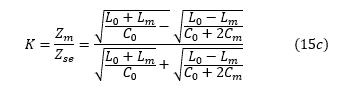‘Zse’ is the single-ended impedance of either of the two lines in the presence of the other line. It is not exactly the same as the single-ended impedance of a single line will be when there is no second line. The presence of the second line somewhat reduces the impedance. The more coupled (or closer) the two lines are, ‘Zse’ will become a little more less.

It is important to note that ‘Zse’ does not change much if the coupling between the two lines is not high. Or if we can make the separation between the two lines greater than the maximum of the conductor width or dielectric height between the signal layer and the closest ground/reference plane.

i.e. ‘Zse’ is relatively the same if the separation is greater than the maximum signal trace width, dielectric height between the signal layer and the nearest reference plane.

Further, ‘Zse’ is not approximate to ’L0/C0’ but is nearer to ’L0/(C0+Cm)’. This may appear surprising at first glance but that is the case.

It is not out of place to define a few new terms: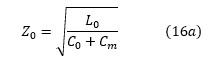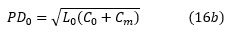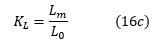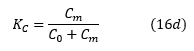‘KL’ and ‘KC’ can be called the inductive and capacitive coupling coefficients respectively. Using these, we can write equation (15) as: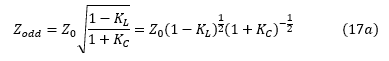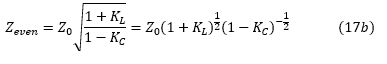Since ‘KL’ and ‘KC’ are lesser than 1, and for most practical cases will be significantly lesser than 1, we can retain only the first order terms so that: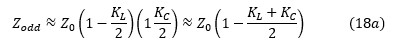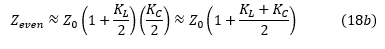If we compare Equation (18a) with (8a) and (18b) with (10a), it is easy to conclude that: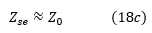And: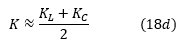It is worth mentioning here that in most practical cases of stripline differential pairs, the inductive coupling coefficient ‘KL’ and the capacitive coupling coefficient ‘KC’ are nearly equal if the dielectric constants of the PCB materials above and below the signal layer are nearly equal.

### Two crosstalk related parameters

Here, we would also like to define two more parameters, NEXT and FEXT: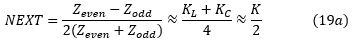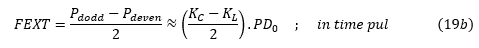NEXT is called the nearest crosstalk coefficient. FEXT is called the far end crosstalk coefficient. These parameters are important in crosstalk analysis when of the two nearby lines, one is the main signal line and the other line is the quiet line on which we wish to determine the crosstalk voltage induced due to signal voltage on the main line. We will develop this point in a next article about crosstalk analysis in detail.

For more information on design rules, check with our DESIGN ASSISTANCE team.